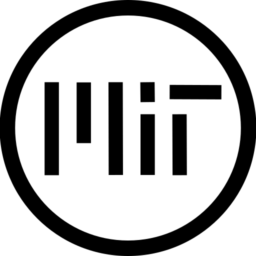# Animated sketchy line

A sketchy outline material for Godot Engine.

This is a multipass shader that works best with 2 passes: the one provided, and an additional one with cull_back and slightly different parameters. Please see the material configuration on itch.io

Warning: does not work well on hard surfaces and meshes with large flat areas.

``````shader_type spatial;

uniform sampler2D outline_noise_tex;
uniform vec4 outline_color:hint_color;
uniform float scissor_value = 0.5;
uniform vec2 uv_scale = vec2(1.0);
uniform sampler2D falloff_curve;
uniform float outline_size = 0.1;
uniform float offset_fres = 0.3;
uniform float fps = 5.0;

varying float h;

void vertex(){
VERTEX += NORMAL * outline_size * texture(outline_noise_tex, UV).r;
h = (WORLD_MATRIX * vec4(VERTEX, 1.0)).y;
}

void fragment(){
float fres = abs(dot(normalize(-NORMAL), normalize(VERTEX)));
vec3 nor = normalize(NORMAL);
float angle = atan(nor.y, nor.x);
angle /= 3.14;

float fres_remap = texture(falloff_curve, vec2(1.0 - fres)).r + offset_fres;
ALPHA = texture(outline_noise_tex, vec2(angle * uv_scale.x + SCREEN_UV.x, nor.z * uv_scale.y + floor(TIME * fps)/fps)).r * fres_remap;
ALBEDO = outline_color.rgb;
ALPHA_SCISSOR = scissor_value;
}``````
###### Tags
multipass, outlineThe shader code and all code snippets in this post are under MIT license and can be used freely. Images and videos, and assets depicted in those, do not fall under this license. For more info, see our License terms.

## More from QbieShay

### Sketchy renderer with optional shadow and tinting.

Subscribe
Notify ofInline Feedbacksgyrosp
2 years ago